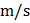# A block of massis released from rest at point. The compression in spring (force constant) when the speed of block is maximum is found to be. What should be the value of?## Question ID - 153900 :- A block of massis released from rest at point. The compression in spring (force constant) when the speed of block is maximum is found to be. What should be the value of?3537

 (4) Speed of block will be maximum whenWhereis compression. SoA block of massis attached to a spring of force-constant. The coefficient of friction between the block and the floor is 0.1. Initially the block is at rest and the spring is un-stretched. An impulse is given to the block as shown in the figure. The block slides a distance ofand comes to rest for the first time. The initial velocity of the block inis. Thenis